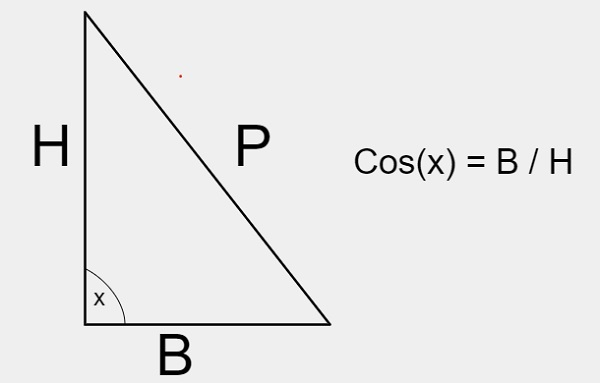# C Program for sum of cos(x) series

We are given with the value of x and n where, x is the angle for cos and n is the number of terms in the cos(x) series.

### For Cos(x)

Cos(x) is a trigonometric function which is used to calculate the value of x angle.### Formula

$$\cos (x) = \displaystyle\sum\limits_{k=0}^\infty \frac{(-1)^{k}}{(2k!)}x^{2k}$$

### For Cos(x) series

Cos(x) = 1 – (x*2 / 2!) + (x*4 / 4!) – (x*6 / 6!) + (x*8 / 8!)……

## Example

Input-: x = 10, n = 3
Output-: 0.984804
Input-: x = 8, n = 2
Output-: 0.990266

Approach used in the below program is as follows

• Input the value of x and n
• Apply the formula for calculating cos(x) series
• Print the result as the sum of all the series

## Algorithm

Start
Step 1 Declare and initialize const double PI = 3.142
Step 2 In function double series_sum(double x, int n)
Set x = x * (PI / 180.0)
Set result = 1
Set s = 1, fact = 1, pow = 1
Loop For i = 1 and i < 5 and i++
Set s = s * -1
Set fact = fact * (2 * i - 1) * (2 * i)
Set pow = pow * x * x
Set result = result + s * pow / fact
End Loop
Return result
Step 3 In function int main() s
Declare and set x = 10
Declare and set n = 3
Print series_sum(x, n)
Stop

## Example

#include <stdio.h>
const double PI = 3.142;
//will return the sum of cos(x)
double series_sum(double x, int n) {
x = x * (PI / 180.0);
double result = 1;
double s = 1, fact = 1, pow = 1;
for (int i = 1; i < 5; i++) {
s = s * -1;
fact = fact * (2 * i - 1) * (2 * i);
pow = pow * x * x;
result = result + s * pow / fact;
}
return result;
}
//main function
int main() {
float x = 10;
int n = 3;
printf("%lf", series_sum(x, n));
return 0;
}

## Output

X=10; n=30.984804
X=13; n=80.974363
X=8; n=2 0.990266